# OECD Researchers data analysis 5 - Simple Linear Regression with one numerical variable in R, ModernDive wayThis post is following of the above post.
In this post, I will do linear regression analysis.

To do this, I make a small(subset) data frame. Let's check what TIME has the most observations.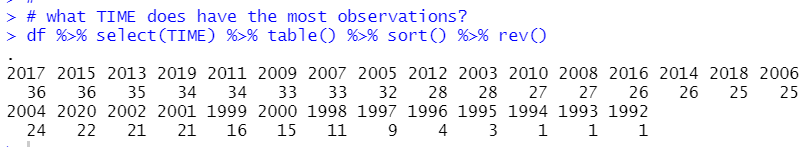2017 and 2015 have 36 observations. So I filter TIME for only 2017 and 2015.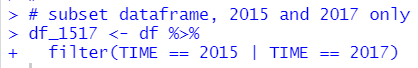Let's see this dataframe with flimpse() function.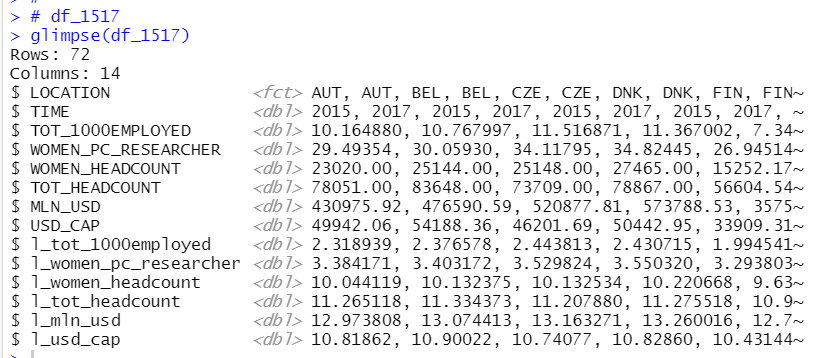I am interested in that "whether Researchers data is related to USD_CAP or l_usd_cap".

So, firstly, let's check correlation.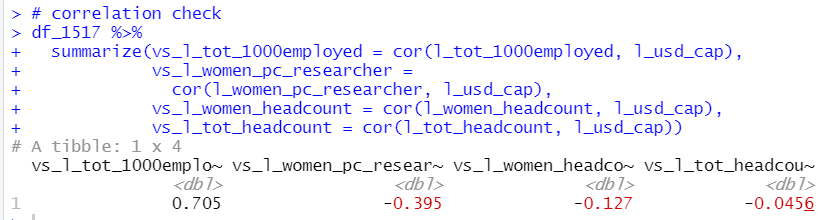I see l_tot_1000employed is the most correlated to l_usd_cap.

So, let's check linear regression, dependent variable = l_usd_cap, independent variable = l_tot_1000_employed.

Before doing linear regression, let's see scatter plot.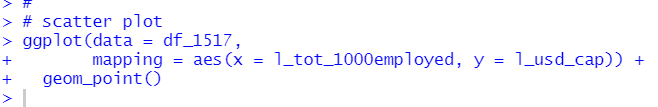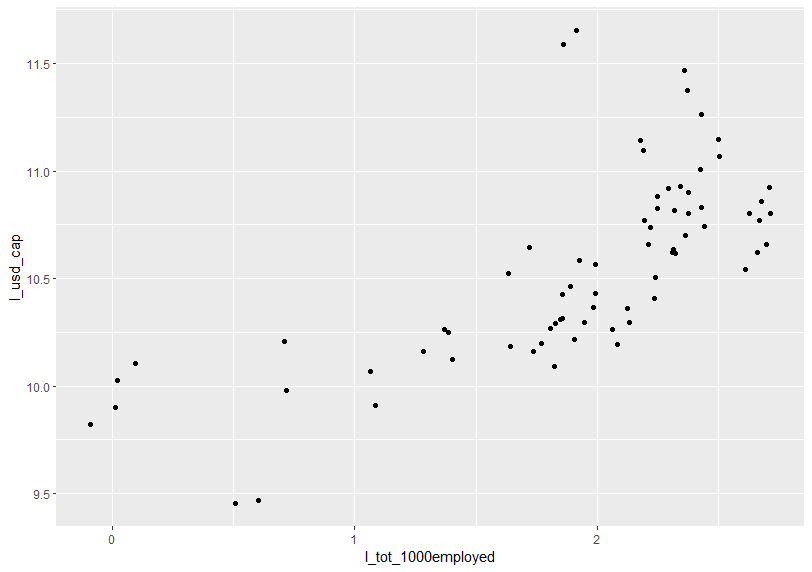I use lm() fundtion to make linear regression object.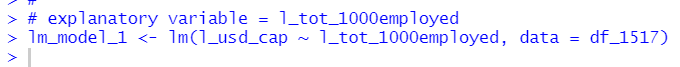Then, I usually use summary() function to see linear regression result, but this time I loaded moderndive package and I will use moderndive packages's get_regression_table() function.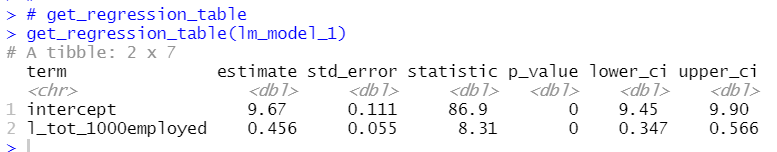I see l_tot_1000employed's estimate is 0.456. It means when l_tot_1000employed increase by 1, l_usd_cap will increase by 0.456. It means when TOT_1000EMPLOYED inclease by 1%, USD_CAP will inclease by 0.456%.

Let's add regression line to the previus scatter plot. I use gerom_smooth() function.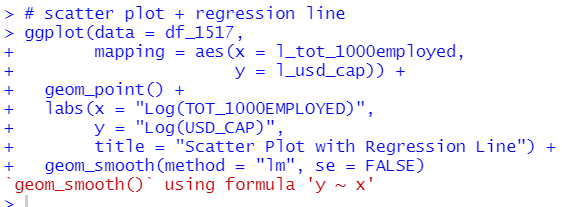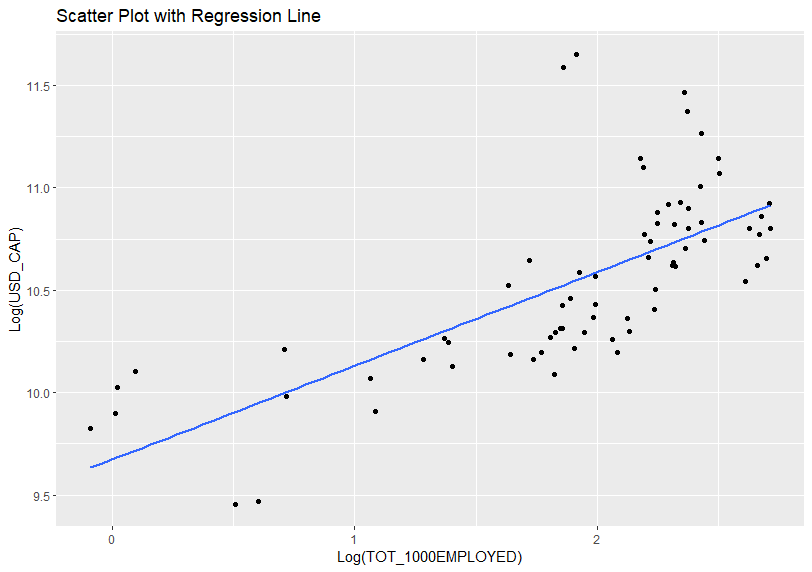That's it. Thank you!

The next post is